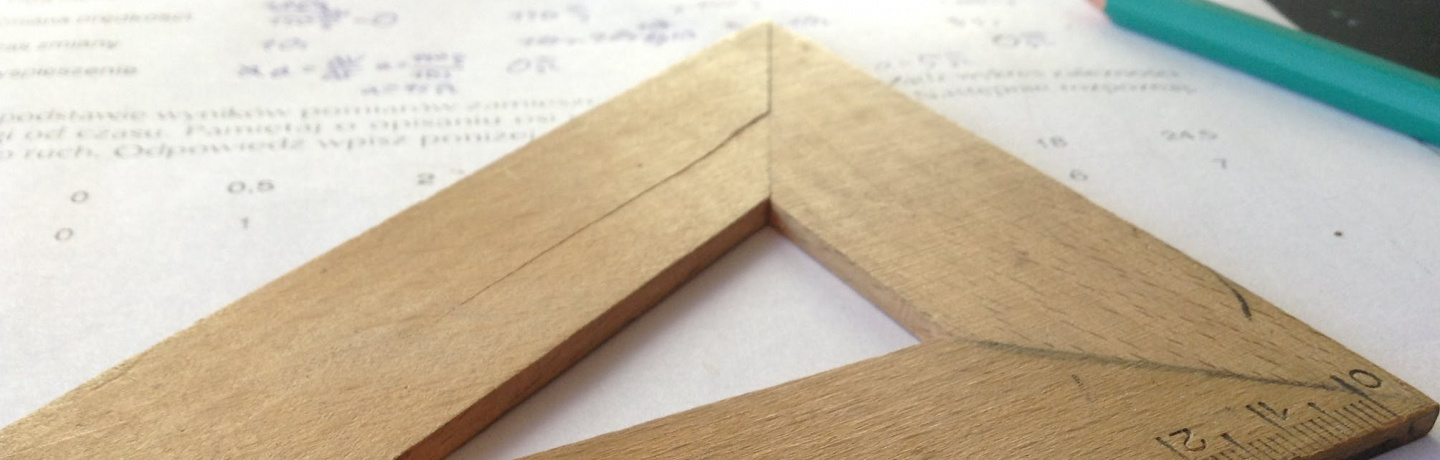# A trace formula problem for foliations

### online | 2020-11-25 | 14:30

Jesús A. Álvarez Lopes

University of Santiago de Compostela, Spain

Let $M$ be a smooth closed manifold, and let $\psi:M\to M$ be a smooth map. A fixed point $p$ of $\psi$ is said to be simple when 1 is not an eigenvalue of the tangent map $\psi_*$ on the tangent space $T_pM$. If all fixed points are simple, then $\psi$ is said to be simple. In this case, the Leftchetz trace formula describes the supertrace of the induced homomorphism $\psi^*$ on the de Rham cohomology $H^*(M)$ using infinitesimal data from the fixed points.

Next, consider a smooth flow $\{\phi^t\}$ on $M$ instead of just a single map. A fixed point $p$ of $\{\phi^t\}$ is simple when it is a simple fixed point of $\phi^t$ for any $t\ne0$. Similarly, a closed orbit $c$ of period $T$ is called simple when, for any $x\in c$, 1 is not an eigenvalue of the map induced by $\phi^T_*$ on the normal bundle of $c$ at $x$. If all fixed points and closed orbits are simple, then $\phi$ is called a simple flow. It would be useful to have version of the Leftchetz trace formula for simple flows, involving infinitesimal data from the fixed points and closed orbits. But it does not make sense because the induced action $\{\phi^{t\,*}\}$ on $H^*(M)$ is trivial (the flow itself defines a homotopy between every $\phi^t$ and the identity map).

Finally, consider also a smooth foliation $\mathcal F$ of codimension one on $M$. It is said that the flow $\{\phi^t\}$ is foliated when every $\phi^t$ maps leaves to leaves. For simple foliated flows, a version of the Leftchetz trace formula was conjectured by Christopher Deninger, using some leafwise version of the de Rham cohomology, and using some supertrace whose values are distributions. The talk will be about our efforts to give appropriate definitions of this leafwise cohomology and distributional supertrace, and to prove this trace formula.

(joint work with Yuri Kordyukov and Eric Leichtnam)